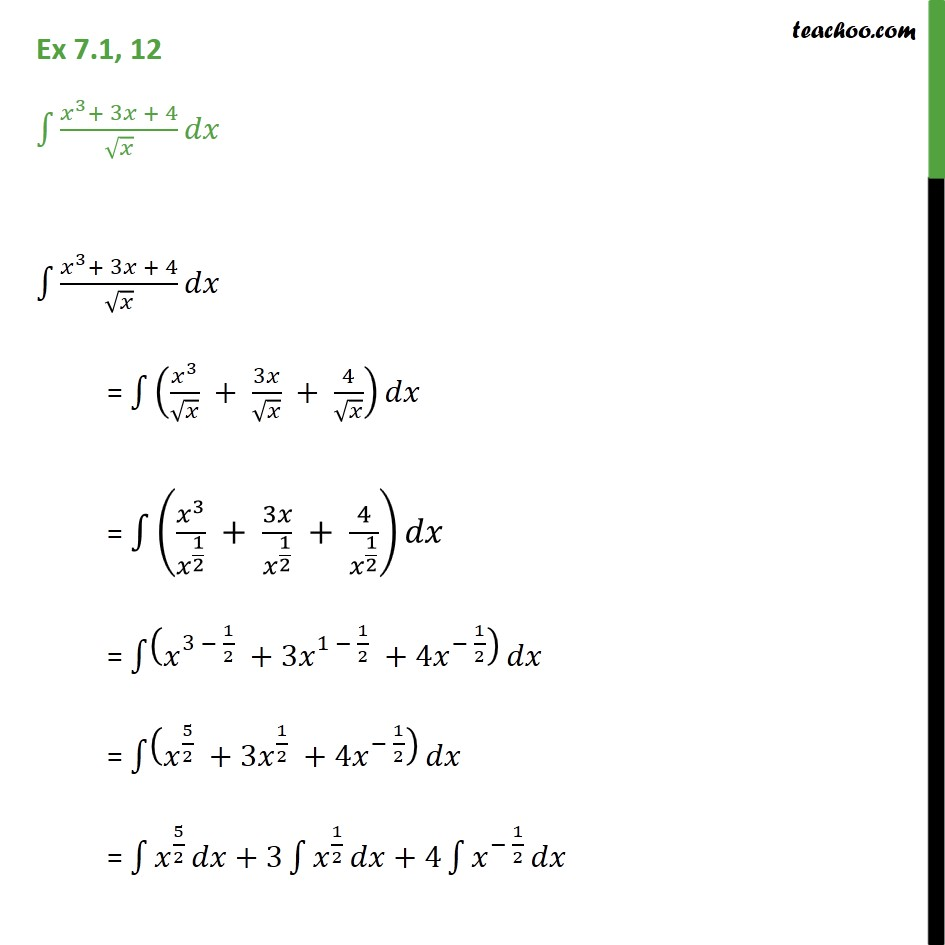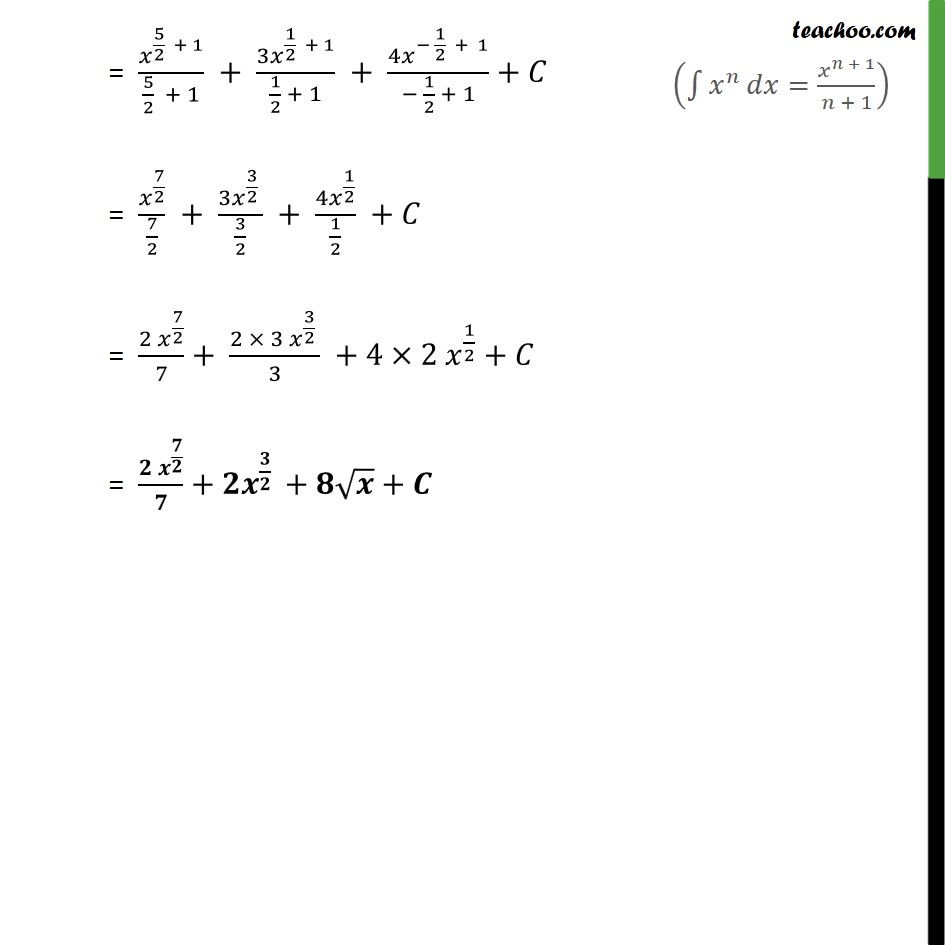1. Chapter 7 Class 12 Integrals (Term 2)
2. Serial order wise
3. Ex 7.1

Transcript

Ex 7.1, 12 3 + 3 + 4 3 + 3 + 4 = 3 + 3 + 4 = 3 1 2 + 3 1 2 + 4 1 2 = 3 1 2 +3 1 1 2 +4 1 2 = 5 2 +3 1 2 +4 1 2 = 5 2 +3 1 2 +4 1 2 = 5 2 + 1 5 2 + 1 + 3 1 2 + 1 1 2 + 1 + 4 1 2 + 1 1 2 + 1 + = 7 2 7 2 + 3 3 2 3 2 + 4 1 2 1 2 + = 2 7 2 7 + 2 3 3 2 3 +4 2 1 2 + = + + +

Ex 7.1

Chapter 7 Class 12 Integrals (Term 2)
Serial order wise